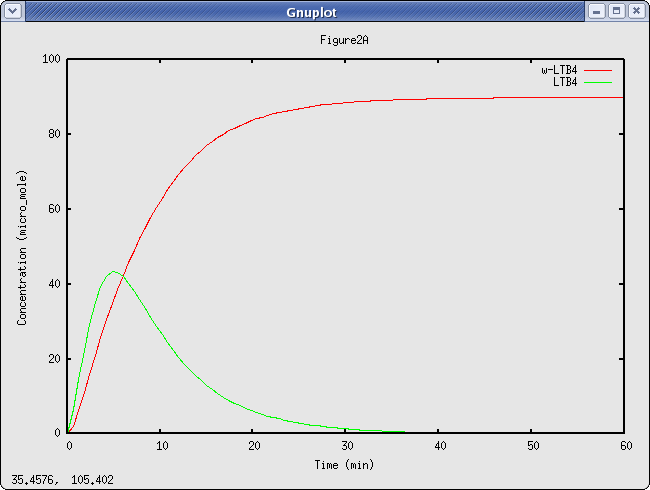## Yang2007_ArachidonicAcidModel Identifier
BIOMD0000000106
Short description

This model is according to the paper Dynamic Simulation on the Arachidonic Acid Metabolic Network . Figure 2A has been reproduced by SBML ode solver on line. In the original model, all the reactions are presented as ODE directly. So curator rewrite each reaction according to the semantics of the paper. In this paper, the authors used quict complex kinetics law to describe the catalysis in the network, curators did not necessarily know all the complete meanings of the paper.

To the extent possible under law, all copyright and related or neighbouring rights to this encoded model have been dedicated to the public domain worldwide. Please refer to CC0 Public Domain Dedication for more information.

In summary, you are entitled to use this encoded model in absolutely any manner you deem suitable, verbatim, or with modification, alone or embedded it in a larger context, redistribute it, commercially or not, in a restricted way or not.

Format
SBML (L2V1)
Related Publication
• Dynamic simulations on the arachidonic acid metabolic network.• Yang K, Ma W, Liang H, Ouyang Q, Tang C, Lai L
• PLoS computational biology , 3/ 2007 , Volume 3 , pages: e55 , PubMed ID: 17381237
• Beijing National Laboratory for Molecular Sciences, State Key Laboratory for Structural Chemistry of Unstable and Stable Species, College of Chemistry and Molecular Engineering, Peking University, Beijing, China.
• Drug molecules not only interact with specific targets, but also alter the state and function of the associated biological network. How to design drugs and evaluate their functions at the systems level becomes a key issue in highly efficient and low-side-effect drug design. The arachidonic acid metabolic network is the network that produces inflammatory mediators, in which several enzymes, including cyclooxygenase-2 (COX-2), have been used as targets for anti-inflammatory drugs. However, neither the century-old nonsteriodal anti-inflammatory drugs nor the recently revocatory Vioxx have provided completely successful anti-inflammatory treatment. To gain more insights into the anti-inflammatory drug design, the authors have studied the dynamic properties of arachidonic acid (AA) metabolic network in human polymorphous leukocytes. Metabolic flux, exogenous AA effects, and drug efficacy have been analyzed using ordinary differential equations. The flux balance in the AA network was found to be important for efficient and safe drug design. When only the 5-lipoxygenase (5-LOX) inhibitor was used, the flux of the COX-2 pathway was increased significantly, showing that a single functional inhibitor cannot effectively control the production of inflammatory mediators. When both COX-2 and 5-LOX were blocked, the production of inflammatory mediators could be completely shut off. The authors have also investigated the differences between a dual-functional COX-2 and 5-LOX inhibitor and a mixture of these two types of inhibitors. Their work provides an example for the integration of systems biology and drug discovery.
Contributors
Kun Yang

is
BioModels Database MODEL8610058649
BioModels Database BIOMD0000000106
isDescribedBy
PubMed 17381237
hasTaxon
Taxonomy Homo sapiens
isVersionOf
isPartOf

Curation status
Curated

Tags
Name Description Size Actions

### Model files

• Model originally submitted by : Kun Yang
• Submitted: Apr 9, 2007 8:28:33 PM
##### Revisions

(*) You might be seeing discontinuous revisions as only public revisions are displayed here. Any private revisionsof this model will only be shown to the submitter and their collaborators.

Legends
: Variable used inside SBML models

Species
Reactions
Reactions Rate Parameters
=> x1; x11, x13, x15, x2, x4 cell*K15*x15*lin*(1+x4/KI19+x2/KI20+x13/KI21+x11/KI22)/(lin+k15*(1+x1/ks)) K15 = 3600.0; KI21 = 500.0; lin = 12.0; ks = 500.0; k15 = 2600.0; KI22 = 500.0; KI19 = 500.0; KI20 = 200.0
x1 => x2; x16 cell*K16*x16*x1/(x1+k16*(1+x2/ks)) K16 = 1000.0; ks = 500.0; k16 = 70.0
x1 => x4; x17, x3 cell*K17*x17*x1/(x1+k17*(1+x4/ki18+x3/ki16+x4/ks)) ks = 500.0; ki18 = 10.0; ki16 = 10.0; k17 = 50.0; K17 = 1000.0
x1 => x6; x18, x7 cell*K18*x18*x1/(x1+k18*(1+x7/ki3+x6/ks)) ks = 500.0; ki3 = 30.0; K18 = 1000.0; k18 = 50.0
x1 => x10; x11, x3, x5, x7, x21 cell*K21*x21*x1/(x1+k21*(1+x5/ki7+x3/ki8+x7/ki11+x11/ki12+x10/ks)) k21 = 5.0; ks = 500.0; ki8 = 4.0; ki7 = 30.0; ki11 = 15.0; K21 = 5000.0; ki12 = 6.3
x1 => 0.1*x1*cell []
x22 => ; x12 cell*K22*x22*x12/((x12+k22)*129) K22 = 125.0; k22 = 20.0
x2 => x3; x24 cell*K24*x24*x2/(x2+k24*(1+x3/ks)) ks = 500.0; k24 = 70.0; K24 = 500.0
x6 => x7; x1, x19, x3 cell*K19*x19*x6/(x6+k19*(1+x1/ki1+x3/ki2+x7/ks)) ks = 500.0; K19 = 3000.0; ki2 = 30.0; k19 = 160.0; ki1 = 0.3
x8 => cell*kd8*x8 kd8 = 0.1
=> x9; x8 kd8*x8*cell kd8 = 0.1
x10 => x11; x24 cell*K24*x24*x10/(x10+k24*(1+x11/ks)) ks = 500.0; k24 = 70.0; K24 = 500.0
x10 => x12; x11, x21, x3, x5, x7 cell*K21*x21*x10/(x10+k21*(1+x5/ki7+x3/ki8+x7/ki11+x11/ki12+x12/ks)) k21 = 5.0; ks = 500.0; ki8 = 4.0; ki7 = 30.0; ki11 = 15.0; K21 = 5000.0; ki12 = 6.3Curator's comment:
(added: 23 Mar 2007, 20:57:51, updated: 23 Mar 2007, 20:57:51)
Figure 2A has been reproduced by SBML ode solver on line.